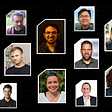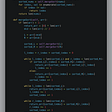# Introduction To Binary Trees

In this short article, I am going to introduce and implement the binary tree data structure. I will also answer questions such as “What is a full binary tree and what is a complete binary tree?”and “How do I traverse a binary tree?”.

First, let’s cover the basic definition of a tree. In computer science, data structures such a arrays, stacks, queues and linked lists are all linear data structures; they differ from trees, which have a hierarchical structure. A tree data structure is composed of nodes; each tree has a root node which has zero or more child nodes and each child node has zero or more child nodes of it’s own. Another important term to know is “leaf node”, which means that it is a node that has no child nodes.

So what is the difference between a tree and a binary tree data structure?

In a binary tree, a node cannot have more than two child nodes.

In a binary tree, a node cannot have more than two child nodes. As you can see on the left in Fig. 1, the root node has three children, therefore it can’t be considered a binary tree. This tree can be called a ternary tree, whereas the tree on the right is a binary tree because each node has no more than two child nodes. So you may be thinking, what is the node composed of? Typically, each node contains some data type as a value; either a string, an integer or another abstract data type.

## Complete Binary Tree

A binary tree is complete if each level is filled (except for the last level) and it is filled from left to right.

A binary tree is complete if each level is fully filled, (except for the last level). If the tree is not fully filled, but it is partially filled from left to right, it is still a complete binary tree. See example below:

## Full Binary Tree

A full binary tree is a tree in which every node has either zero or two children.

A full binary tree is a tree in which every node has either zero or two children. An easy way to remember this one is that if one of the nodes only has one child, it is not a full binary tree. In Figure 3 below, the binary tree on the left is not a full tree because it has two nodes that only have one child node whereas in the tree on the right is considered full because each of it’s nodes has either two or no child nodes.

## Perfect Binary Tree

A binary tree is perfect if it is both full and complete.

A binary tree is perfect if it is both full and complete. All the leaf nodes will be at the same level and as a result, this level has the largest number of nodes.

Now that we have our terminology covered, let’s jump into the code. I will be using Python to implement a simple binary tree with an insertion method.

In the example above, we are implementing a binary tree that follows a particular ordering; all the nodes are ordered from left to right.

In the __init__ method, we define the initialization details of our node. Since binary tree nodes cannot have more than two children, each node contains a variable that points to the left child node and the right child node (as well as the value it holds).

Each time a value is inserted into our tree, the insert method compares the value of the node to the parent node. If the value that is being added is smaller then the parent, than it will be added to the left. And if the value being added is larger than the parent node, than it will be added to the right.

If the child node does not exist (the child node is equal to None) than a new node is created with that value. (eg: self.left = Node(val) ). However, if there is a child node in place than the insert method is recursively called on that child node.

The following gif from Penjee.com visually explains what happens when we insert values using the code in Figure 5.

I hope this short introduction gives you a rough idea of the basics of binary trees!

Happy Coding!

--

--

--

## More from Anton Suprun

Computer Science Student, Programming Enthusiast

Love podcasts or audiobooks? Learn on the go with our new app.

## Recommended from Medium## Move Fast And Don’t Break Things## How a Googler solves coding problems## 12 Java Developers to Follow## Wrong Ways of Defining Service Boundaries## Anton Suprun

Computer Science Student, Programming Enthusiast

## Leetcode Patterns Adventure 2  — Missing Number## [LeetCode]#2053. Kth Distinct String in an Array